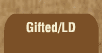Mathematics and Dyslexia

Tips for Learning the Times Tables

Tools for the Times Tables & Workbook

Home > Math > times tables practice > Sevens (low, in order)

## The sevens Times Tables - first half in order

No tricks for making sevens easier, except that its 5 + 2, or 6 + 1... so 7 x 5 would be the same as 6 x 5(30) +1 x 5 (5) to give you 35.

1. 7 x 0=
2. 7 x 1=
3. 7 x 2=
4. 7 x 3=
5. 7 x 4=
6. 7 x 5=
7. 5 x 7=
8. 4 x 7=
9. 3 x 7=
10. 2 x 7=
11. 1 x 7=
12. 4 x 7=
13. 7 x 3=
14. 2 x 7=
15. 3 x 7=